• 使用下面的代码可以初始化一个大小为n的一维数组 vector<int> dp(n); 二维数组 使用下面的代码可以初始化一个row 行col列的二维数组 vector<vector<int>> dp(row, vector<int>(col)); 三...

一维数组

使用下面的代码可以初始化一个大小为n的一维数组

vector<int> dp(n);

二维数组

使用下面的代码可以初始化一个row 行col列的二维数组

vector<vector<int>> dp(row, vector<int>(col));

三维数组

使用下面的代码可以初始化一个三维数组，维度分别为n1, n2, n3

vector<vector<vector<int>>> dp(n1, vector<vector<int>>(n2, vector<int>(n3)));

展开全文• vector<vector<double>> w(10,vector<double>(10,-1)); 说明: 创建 10x10 二维数组, 初始值为 -1.0.
vector<vector<double>> w(10,vector<double>(10,-1));

说明:
创建 10x10 二维数组, 初始值为 -1.0.

展开全文c++
• 一、vector初始化 (1) vector<int> a(10); //定义了10个整型元素的向量（尖括号中为元素类型名，它可以是任何合法的数据类型），但没有给出初值，其值是不确定的。 测试 #include<iostream> #include...

vector()的用法
概念
vector 是向量类型，它可以容纳许多类型的数据，如若干个整数，所以称其为容器。vector 是C++ STL的一个重要成员，使用它时需要包含头文件：

#include<vector>;

一、vector的初始化

(1) vector<int> a(10); //定义了10个整型元素的向量（尖括号中为元素类型名，它可以是任何合法的数据类型），但没有给出初值，其值是不确定的。

测试

#include<iostream>
#include<math.h>
#include<vector>
using namespace std;

int main()
{
vector<int> a(10);

for(int i=0;i<a.size();i++)
cout<<a[i]<<" ";
return 0;
}

结果：(感觉默认生成为0)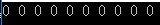2）vector<int> a(10,1); //定义了10个整型元素的向量,且给出每个元素的初值为1

测试

#include<iostream>
#include<math.h>
#include<vector>
using namespace std;

int main()
{
vector<int> a(10,1);

for(int i=0;i<a.size();i++)
cout<<a[i]<<" ";
return 0;
}

结果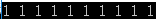3）vector<int> a(b); //用b向量来创建a向量，整体复制性赋值

测试：

#include<iostream>
#include<math.h>
#include<vector>
using namespace std;

int main()
{
vector<int> b(10,3);
vector<int> a(b);

for(int i=0;i<a.size();i++)
cout<<a[i]<<" ";
return 0;
}

结果：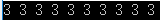测试：

#include<iostream>
#include<math.h>
#include<vector>
using namespace std;

int main()
{
vector<int> b;
for(int i=0;i<10;i++)
b.push_back(i);
vector<int> a(b.begin(),b.begin()+3); //定义了a值为b中第0个到第2个（共3个）元素

for( i=0;i<a.size();i++)
cout<<a[i]<<" ";
return 0;
}

结果：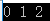5int b={1,2,3,4,5,9,8};
vector<int> a(b,b+7); //从数组中获得初值 从b开始 一共7个

测试：

#include<iostream>
#include<math.h>
#include<vector>
using namespace std;

int main()
{
int b={1,2,3,4,5,6,7};
vector<int> a(b,b+7); //从数组中获得初值 从b开始 一共7个！！！！

for( int i=0;i<a.size();i++)
cout<<a[i]<<" ";
return 0;
}

结果：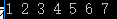二、vector对象的几个重要操作，举例说明如下：

1）a.assign(b.begin(), b.begin()+3); //b为向量，将b的0~2个元素构成的向量赋给a2）a.assign(4,2); //是a只含4个元素，且每个元素为23）a.back(); //返回a的最后一个元素4）a.front(); //返回a的第一个元素5）a[i]; //返回a的第i个元素，当且仅当a[i]存在6）a.clear(); //清空a中的元素7）a.empty(); //判断a是否为空，空则返回ture,不空则返回false8）a.pop_back(); //删除a向量的最后一个元素9）a.erase(a.begin()+1,a.begin()+3); //删除a中第1个（从第0个算起）到第2个元素，也就是说删除的元素从a.begin()+1算起（包括它）一直到a.begin()+         3（不包括它）10）a.push_back(5); //在a的最后一个向量后插入一个元素，其值为511）a.insert(a.begin()+1,5); //在a的第1个元素（从第0个算起）的位置插入数值5，如a为1,2,3,4，插入元素后为1,5,2,3,412）a.insert(a.begin()+1,3,5); //在a的第1个元素（从第0个算起）的位置插入3个数，其值都为513）a.insert(a.begin()+1,b+3,b+6); //b为数组，在a的第1个元素（从第0个算起）的位置插入b的第3个元素到第5个元素（不包括b+6），如b为1,2,3,4,5,9,8         ，插入元素后为1,4,5,9,2,3,4,5,9,814）a.size(); //返回a中元素的个数；15）a.capacity(); //返回a在内存中总共可以容纳的元素个数16）a.resize(10); //将a的现有元素个数调至10个，多则删，少则补，其值随机17）a.resize(10,2); //将a的现有元素个数调至10个，多则删，少则补，其值为218）a.reserve(100); //将a的容量（capacity）扩充至100，也就是说现在测试a.capacity();的时候返回值是100.这种操作只有在需要给a添加大量数据的时候才         显得有意义，因为这将避免内存多次容量扩充操作（当a的容量不足时电脑会自动扩容，当然这必然降低性能） 19）a.swap(b); //b为向量，将a中的元素和b中的元素进行整体性交换20）a==b; //b为向量，向量的比较操作还有!=,>=,<=,>,<

三、顺序访问vector的几种方式，举例说明如下：

（1）向向量a中添加元素

1、利用push_back()

vector<int> a;
for(int i=0;i<10;i++)
a.push_back(i);

2、也可以从数组中选择元素向向量中添加

int a={1,2,3,4,5,6};
vector<int> b；
for(int i=1;i<=4;i++)
b.push_back(a[i]);

3、也可以从现有向量中选择元素向向量中添加

int a={1,2,3,4,5,6};
vector<int> b;
vector<int> c(a,a+4);
for(vector<int>::iterator it=c.begin();it<c.end();it++)
b.push_back(*it);

4、也可以从文件中读取元素向向量中添加

ifstream in("data.txt");
vector<int> a;
for(int i; in>>i)
a.push_back(i);

5、【误区】

vector<int> a;
for(int i=0;i<10;i++)
a[i]=i;

//这种做法以及类似的做法都是错误的。刚开始我也犯过这种错误，后来发现，下标只能用于获取已存在的元素，而现在的a[i]还是空的对象

（2）从向量中读取元素

1、通过下标方式读取

int a={1,2,3,4,5,6};
vector<int> b(a,a+4);
for(int i=0;i<=b.size()-1;i++)
cout<<b[i]<<" ";

2、通过遍历器方式读取

int a={1,2,3,4,5,6};
vector<int> b(a,a+4);
for(vector<int>::iterator it=b.begin();it!=b.end();it++)
cout<<*it<<" ";

四、几种重要的算法，使用时需要包含头文件：

#include<algorithm>1sort(a.begin(),a.end()); //对a中的从a.begin()（包括它）到a.end()（不包括它）的元素进行从小到大排列2reverse(a.begin(),a.end()); //对a中的从a.begin()（包括它）到a.end()（不包括它）的元素倒置，但不排列，如a中元素为1,3,2,4,倒置后为4,2,3,13copy(a.begin(),a.end(),b.begin()+1); //把a中的从a.begin()（包括它）到a.end()（不包括它）的元素复制到b中，从b.begin()+1的位置（包括它）开        始复制，覆盖掉原有元素4find(a.begin(),a.end(),10); //在a中的从a.begin()（包括它）到a.end()（不包括它）的元素中查找10，若存在返回其在向量中的位置

展开全文c++
• vector<int> a(nums.begin(), nums.end()); 注意：vector<int> a(nums.begin()+1, nums.end()-2);中a包含nums.begin()+1，不包含nums.end()-2，也就是包含的区间是左闭右开区间

vector<int> a(nums.begin(), nums.end());

注意：vector<int> a(nums.begin()+1, nums.end()-2);中a包含nums.begin()+1，不包含nums.end()-2，也就是包含的区间是左闭右开区间

如果a不能在声明的时候初始化，可以先定义再初始化：

vector<int> a;
a=vector<int>(nums.begin(), nums.end());

展开全文• 刷题中遇到需要初始化一个vector二维数组，使用两个for已经不适用了，， 特此记录vector构造函数创建二维数组 //初始化一个m行n列的元素值全为0的二维数组 vector<vector<int>> matrix (n, vector<...
• c++vector初始化错误第一篇文章，记一下刷题遇到的坑 第一篇文章，记一下刷题遇到的坑 leetcode 303要求一个数组i到j的和，我想用一个数组v记录.v[i]表示从num到num[i] (包含二者)的和，然后计算的时候用v[j...c++ leetcode 数据结构
• 默认初始化，最常用 此时，vector为空， size为0，表明容器中没有元素，而且 capacity 也返回 0，意味着还没有分配内存空间。 这种初始化方式适用于元素个数未知，需要在程序中动态添加的情况。 2.vector<int&...c++ 算法 链表
• 数组初始化方式： 2.vector六种初始化方式： 3.string初始化方式： 4.string也能push_back()和pop_back() 5.string的插入删除操作 1.数组初始化方式： https://www.cnblogs.com/yyzyxy/p/10336480.html 2.vector六种...c++
• #include <...//法一：自己写for循环进行初始化分配空间 void Init1(int m, int n) { vector<vector<int>> test; test.resize(m); for (int i = 0; i < m; i++) { test[i].resize(n);c++ vector
• int countOdds(int low, int high) { ... std::vector<int>temp{high-low+1,0}; int n = low; std::generate(temp.begin(),temp.end(),[&]{return n++;}); for (auto x:temp) { std::cout <...
• 转载 #include<iostream> #include <vector> int main() { //1. 不带参数的构造函数初始化 vector<... //2.... //初始化了10个默认值为0的元素 vector<int> cde(10, 1); //初vector c++
• 在某些情况下，初始化的真实含义依赖于传递初始值时用的是花括号还是圆括号，例如，用一个整数来初始化vector<int>时，整数的含义可能是vector对象的容量也可能是元素的值。类似的，用两个整数来初使化vector&...c++ 开发语言 后端
• //定义的同时初始化大小 vector<int> vector_1D_3(n,m);//定义的同时初始化大小为n,元素初始值为m //先定义变量，再初始化大小和初值 vector<int> vector_1D_4; vector_1D_4=vector<int>(n,m); ...c++ vector
• 我自己写的，学习数据结构和算法的时侯经常需要产生一定规模的随机数据进行测试(比如比较各种排序算法)，所以写了这些工具函数，用于产生随机数的vector，以及复制和输出vector。大部份是在windows里用Code::Blocks...c语言随机函数初始化
• 初始化一个vector<complex> void main() { vector < complex < double >> a = { {1,2},{3,4},{5,6} }; // 运行成功 注意里面每个complex都要有大括号 } 初始化一个vector<vector&gc++
• C++：vector二维数组初始化

千次阅读 2021-03-31 15:12:37
在刷 LeetCode 的时候需要初始化创建一个行大小为r、列大小为c的 vector 二维数组，具体的实现方法如下： vector<vector<int>> num(r, vector<int>(c)); 初始化创建r个类型为vector<int>，...c++ 开发语言
• 初始化 vector<int>ivec1(10,42); //内置方法，初始化的内容为10个42 vector<int>ivec2(10); vector<int>::size_type ix=0; for(ix;ix<10;++ix) { ivec2[ix]=42; //下标操作 }...c++
• template vector<vector> my_matrix(size_x, vector(size_y));
• 如果使用vector的resize()之前其大小(size()==0)为0,则resize()会给每个初始化；如果之前大小不为0，则之前部分的值不变，后面扩充的空间的值会初始化。所以，如果第二种情况下希望也能全部初始化，则可以先clear()...
• vector常用方法assign() 对Vector中的元素赋值void assign( input_iterator start, input_iterator end ); //void assign( size_type num, const TYPE &val );reserve() 设置Vector最小的元素容纳数量 函数为...
• 初始化二维vector，为r*c的vector，所有值为0. 直接用初始化方法 vector<vector > newOne(r, vector(c, 0)); 用resize()来控制大小 vector<vector<int> > res; res.resize(r);//r行 for ...
• 假设dps是一个m×n维的vector，则初始化dps为全零的方法为： vector<vector<int>> dps(m, vector<int>(n));
• c++使用vector创建二维0矩阵（初始化） 一、前言 c++真的是又爱又恨的一门语言，本人是一名python程序员但是因工作需求不得不投入到c++的大军中，逐渐偏离人生苦短我用python的初衷，唠叨完毕开始正题。 最近在做一...
• 目录vector初始化at函数clear函数 vector初始化 vector初始化有很多，以下列举几种。 vector<int> v; //定义一个vector容器，类型是int，size为0 vector<int> v = {0,1,2}; //定义一个vector容器...c++ 容器 开发语言
• ※原因分析：使用memset初始化树节点时，vector被使用错误方式初始化 事件梗概： ①背景：今天在练习写A星寻路的时候发现程序异常结束。 图1：异常结束的画面 ②于是进行调试发现是vector里面炸了，具体代码如下图...c++
• vector初始化与清空 vector初始化 vector <int> v; 如果直接进行访问 v[i] 会报错 解决方案： 可以使用 v.resize(n)或者v.resize(n, m) 来初始化，前者是使用n个0来初始化，后者是使用n个m来初始化...c++
• vector (1): vector<int> list； (2): vector<int> ilist2(ilist); vector<int> ilist2 = ilist; (3): vector<int> ilist = {1,2,3.0,4,5,6,7}; vector<int> ilist {1,2,3.0,4,...c++
• 除了用大括号直接赋值，还可以用小括号指明大小和全部初始值 #include<iostream> #include<... // vector初始化 for (int num:ans){ cout<<num<<" "; } return 0; } ...c++
• vector<vector<double>> dist;//distance list for (i = 0; i < n; i++) {//init distance list for (j = 0; j < n; j++) { dist[i].push_back(0.1); } } 分析原因后觉得应该是vector<...c++ 开发语言
• 一维 vector<int>(列数, 0); 二维 vector<vector<int>> newOne(行数, vector<int>(列数, 0));c++...

c++vector初始化c++ 订阅